#### Theory of Static Characteristics I and Bipolar Transistor Switch

The Ideal Inverter:

Prior to examining the performance of bipolar transistor inverter, it is helpful to have knowledge of what the needs of an ideal inverter are. The gate is predicted to accept an input logic signal in defined voltage levels, invert it and give an output voltage to drive other gates as shown in figure below.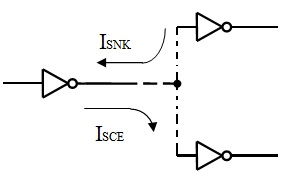Figure: The Ideal Inverter

a) Logic Voltages: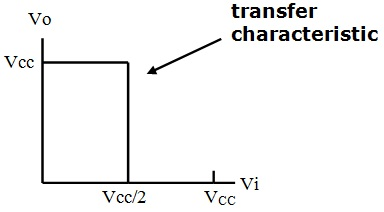logic HI  VH = VCC
logic LO VL = 0V
If Vi < VCC/2, VO = VH = VCC
If Vi > VCC/2, VO = VL = 0V

(b) Drive Capability:

Ideally ISNK, ISCE → ∞

VO must be independent of load conditions

(c) Noise Immunity: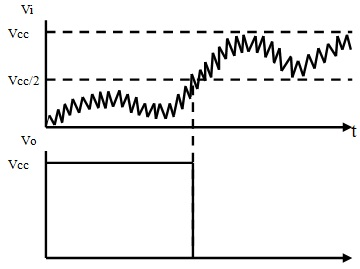The output must be insensitive to noise superimposed on the input and must provide defined switching.

d) Switching Speed: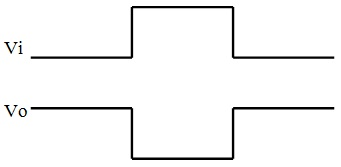Ideally, there must be no switching delay however an instantaneous response of the output to a change at input.

Bipolar Transistor Switch
:

Basically, the bipolar transistor switch shown in figure below functions in one or other of two states.

OFF state – Transistor in the Cut-Off mode.
ON state – Transistor in the Saturation mode.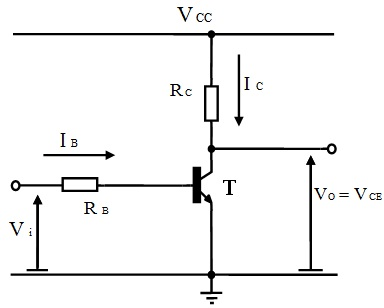Figure: A Simple Bipolar Transistor Inverter

Base resistor RB, serves to control the level of base current. The collector resistor RC, serves to limit the maximum collector current however acts basically as an output load for the transistor.

Figure below shows a set of output characteristics for the transistor as IC vs. VCE for the range of values of IB. The load line can be superimposed on such curves which states the operating path followed by the output of transistor for particular load resistor, RC and supply voltage VCC. This path is set up as follows:

Load line: IC = (VCC - VCE)/RC = - [(1/RC) VCE] + (VCC/RC); the equation of a straight line.

If IC = 0 then, VCE/RC = VCC/RC => Vo = VCE = VCC

If VCE = 0 then, IC = VCC/RC = IC max

Such points can be employed to plot the load line superimposed on the characteristic curves of transistor. Figures below show the contrast between operating the transistor as an amplifying device in forward active region and operating it as a logic switch where it consists of two states; either ON in saturation mode or OFF in the cut-off mode.

(i) Operation as an Amplifier:

Whenever operating in linear active region, base emitter junction is forward biased and the base collector junction is reverse biased. Beneath such conditions:

IC = βF IB and Vo = VCE = VCC - ICRC

This can be seen that a small signal superimposed on base current is amplified to provide a much greater change on collector current. The variation in collector current, passing via the load resistor, RC, transforms this into an output voltage signal. Note that, as the IC rises, VO reduces and vice-versa and for this reason the signal is inverted from input to output.

(ii) Operation as a Switch:

Whenever acting as a switch, the transistor operates exterior of the linear active region beneath steady-state conditions and only passes via it when changing the state. As a switch, the transistor functions either in cut-off region or the saturation region.

Cut-Off:

With Vi = 0,   VBE = 0,   IB = 0, then IC = 0,   Transistor is OFF

Then VO = VCC – ICRC = VCC

Therefore, with Vi = 0 = VL   input LO, we encompass VO = VCC = VH output HI

This is the logic inverting action.

Saturation:

In linear active region, IC = βF IB. Whenever using the transistor as a switch, the load resistor RC, is employed as a current limiting resistor to limit the collector current to IC = IC max = VCC/RC. When a high base current is injected to the transistor in such a way that:

βF IB >> ICMAX   that is, IB >> ICMAX/ βF

Then the transistor can’t amplify the base current, IB, since the potential resultant current can’t flow in the collector, as the collector current is restricted to IC max. In this case, the transistor can’t carry on executing in the forward active region and is driven into saturation region. Here with:

Vi = Vcc , IB = (VCC - VBE)/RB  then IC = ICMAX = (VCC - VCESAT)/RC

The Transistor is ON and Vo = VCE SAT → 0 usually 0.1 – 0.2V

Hence with,

Vi = VCC = VH input HI we have, VO = VCESAT = VL output LO

This is as well as logic inverting action.

In saturation, the base of transistor is stated to be overdriven. That is to state more current is fed into the base than is needed to produce the maximum current which can flow in the collector. Usually, 4 to 5 times the current required to bring the collector current to IC max is injected to the base to guarantee that it is overdriven and the transistor executes in the saturation mode.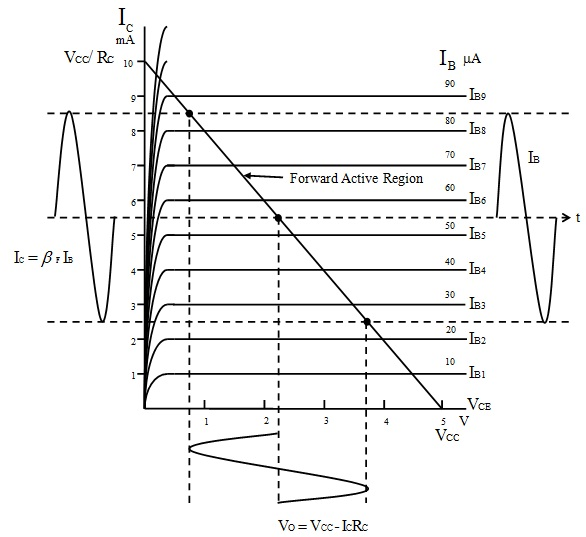Figure: Operation of the Bipolar Transistor as an Amplifier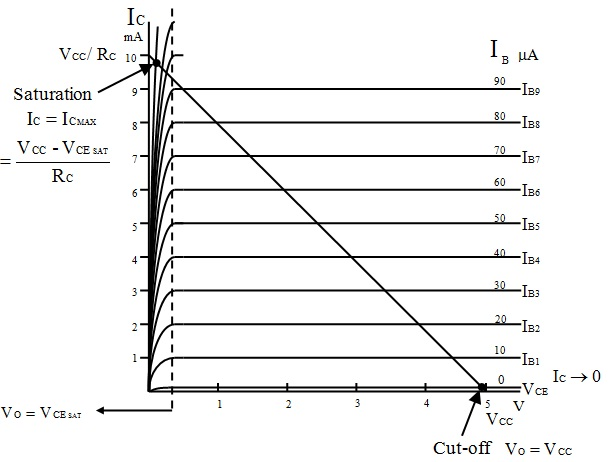Figure: Operation of the Bipolar Transistor as a Switch

Latest technology based Electrical Engineering Online Tutoring Assistance

Tutors, at the www.tutorsglobe.com, take pledge to provide full satisfaction and assurance in Electrical Engineering help via online tutoring. Students are getting 100% satisfaction by online tutors across the globe. Here you can get homework help for Electrical Engineering, project ideas and tutorials. We provide email based Electrical Engineering help. You can join us to ask queries 24x7 with live, experienced and qualified online tutors specialized in Electrical Engineering. Through Online Tutoring, you would be able to complete your homework or assignments at your home. Tutors at the TutorsGlobe are committed to provide the best quality online tutoring assistance for Electrical Engineering Homework help and assignment help services. They use their experience, as they have solved thousands of the Electrical Engineering assignments, which may help you to solve your complex issues of Electrical Engineering. TutorsGlobe assure for the best quality compliance to your homework. Compromise with quality is not in our dictionary. If we feel that we are not able to provide the homework help as per the deadline or given instruction by the student, we refund the money of the student without any delay.

2015 ©TutorsGlobe All rights reserved. TutorsGlobe Rated 4.8/5 based on 34139 reviews.